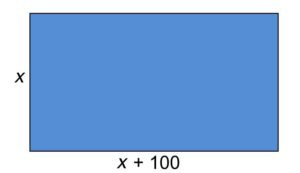## Putting It Together: Equations and Inequalities

At the beginning of this module, we explored a few problems associated with the construction of a school. First we had to set up a mathematical model that would accurately reflect the following requirements for the foundation of the building.

• The shape must be rectangular.
• The length must be 100 ft. longer than the width.
• There must be a total of 120,000 sq. ft. in the foundation.Letting $x$ stand for the width of the rectangle, the length must then be $x+100$. The area of any rectangle is equal to the product of its length and width, and since the area must be 120,000 sq. ft., this leads to the equation,

$\left(x+100\right)\left(x\right)=120,000$

$x^2+100x=120,000$

$x^2+100x-120,000=0$

The equation is quadratic, that is, degree 2.  In this case, the trinomial can be factored.

$\left(x-300\right)\left(x+400\right)=0$

This implies either $x=300$, or $x=-400$. However, since negative width does not make much sense (have you ever tried to order a negative 8 foot fence post?), we only use the positive answer, $x=300$. This means the width is 300 ft., and the length is 300 + 100 = 400 ft.  A quick check verifies that the dimensions yield the correct square footage:

$300\times400=120,000$

Ok, now that we have our foundation planned, let’s move on to the walls. Recall, this project requires at least 1800 concrete blocks.  The budget for this material is $2,400, and each block costs$1.20.  Furthermore, there is a fee of \$100 for hauling the blocks to the construction site, regardless of how many blocks are ordered.

Let $n$ stand for the number of blocks that would be purchased. Then two inequalities can be set up based on the constraints in the problem.

$n\ge1800$

$(1.20)n+100\le2400$

What is the maximum number of blocks that you can purchase? Let’s solve the second inequality to find out.

$(1.20)n\le2400-100=2300$

$n\le{\Large\frac{2300}{1.20}}\approx1916.7$

There is no use for a fraction of a block, so we might as well say that $n\le1916$. A maximum of 1916 whole blocks can be purchased. Notice that $1916\ge1800$, so the first inequality is also satisfied, meaning that our budget allows for more than enough blocks to complete this phase of the project.

With the help of equations and inequalities, as well as specialized techniques for solving them, the plans for the new school are coming together nicely!